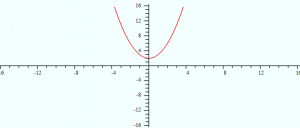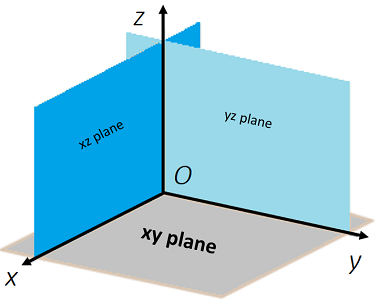# Analytic Geometry

Analytic geometry creates a connection between graphs and equations. For example, the linear function f(x) = x2 – 2 (an equation) can also be represented by a graph:Euclidean Geometry is based solely on geometric axioms without formulas or co-ordinates; Analytic geometry is the “marriage” of algebra and geometry with axes and co-ordinates .

## Calculus and Analytic Geometry

Calculus and analytic geometry have become so intertwined, it’s rare nowadays to find a course in pure “Analytic Geometry”. It’s more common to take a course in Calculus and Analytic Geometry, which blends the principles of basic analytic geometry with concepts like functions, limits, continuity, derivatives, antiderivatives, and definite integrals.

Curves:

## X Y Plane

In 3D space (also called xyz space), the xy plane contains the x-axis and y-axis:The xy plane can be described as the set of all points (x, y, z) where z = 0. In other words, any point (x, y, 0). For example, all of the following points are on the xy plane:

• (1, 5, 0)
• (-2, 19, 0)
• (π, -1, 0)
• (.5, .2, 0)

This fact gives us the equation for the xy plane: z = 0.

This is just an extension of the same idea of the x-axis (in the Cartesian plane) being the place where y = 0:(Left) The x-axis in 2D is where points are located at y = 0. In 3D, the xy plane has points where z = 0.

The xy plane, together with the yz plane and xz plane, divide space into eight octants. The O in the center of the diagram is the origin, which is a starting point for the 3D-coordinate system. The points are described by an ordered triple of real numbers (x, y, z). For example, the point (2, 3, 0) can be found at:

• x = 2,
• y = 3,
• z = 0.

As z is zero, we know this point must be somewhere on the xy plane.

## Distance Formula for Points in the XY Plane

The distance between any two points in xyz-space can be found with a generalization of the distance formula:Example question: What is the distance between the points (4, 3, 0) and (2, 9, 0)?

Step 1: Identify the coordinate components that we need to put into the formula. We know our coordinates are always ordered (x, y, z), so:

1. (4, 3, 0):
• x1 = 4
• y1 = 3
• z1 = 0.
2. (2, 9, 0):
• x2 = 2
• y2 = 9
• zz = 0.

Don’t worry about which coordinate is which (e.g. does x = 4 go into x1 or x2?). The distance formula squares these values, so you’ll get the same answer no matter which way you choose.

Step 2: Plug your values from Step 1 into the distance formula:If you aren’t good with algebra, head over to Symbolab and just replace the x, y, z values with your inputs.

A quadric surface is a 3D extension of a conic (ellipsis, hyperbola, or parabola). In 2D space, they are defined by quadratic equations in 2D space . Quadric refers to the degree of the equation describing the surface: variables in these equations are raised to the 2nd power. They can be described, in general, as a graph of an equation expressed in the form :

Ax2 + B2 + C2 + Dxy + Exz + Fyz + Hx + Iy + Jz + K = 0.

Where A, B, C, D, E, F, H, I, J, K are fixed constants and x, y, z, are variables.

Different types of quadric surfaces can be obtained from simplifying this equation by rotations and translations of the x, y, z coordinate axes.There are six basic types of quadric surface :

1. Ellipsoid,
2. Elliptic paraboloids,
3. Hyperbolic paraboloid,
4. Cones,
5. Hyperboloids of one sheet,
6. Hyperboloids of two sheets.

Other examples of quadratic surfaces include the cylinder, elliptic cone, elliptic cylinder, elliptic hyperboloid, hyperbolic cylinder, paraboloid, sphere, and spheroid.

1. EllipsoidThe general equation for an ellipsoid is:If A = B = C, the shape is a sphere.

2. Elliptic paraboloidsThe equation for an elliptic paraboloid is:Elliptic paraboloids have ellipses as cross sections. If A = B, the cross section is a circle.

3. Hyperbolic paraboloid

The equation for a hyperbolic paraboloid is:Hyperbolic paraboloids are saddle-shaped, like a Pringle. The sign of c determines whether the graph opens up or down. A positive value for c has the saddle right side up (as if it were being placed on a horse).

4. Cones
The equation for a cone is :5. Hyperboloids of one sheet
The equation for the hyperboloid of one sheet is:6. Hyperboloids of two sheets
The equation for the hyperboloid of two sheets is:## References

 Analytic Geometry and Calculus. Retrieved May 3, 2021 from: math.uci.edu/~ndonalds/math184/analytic.pdf